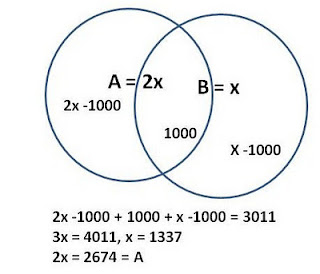## Saturday, September 1, 2018

### 2011 Mathcounts Chapter Sprint Round solutions#22: The answer is 2674.
See left for explanations.

#23: Let the two consecutive positive integers be x and x + 1.
( x + 1 ) / x = 1.02, x + 1 = 1.02x, 0.02x = 1, x = 1 divided by 0.02 = 1 times 100/2 = 50
The two numbers are 50 and 51 and their sum is 50 + 51 = 101.

#24: The two x-intercepts when y is "0" are 10 or -10; the two y-intercepts when x is "0" are 5 or -5.
Area of a rhombus is D1 x D2 / 2 so the answer is [10-(-10)] x [5 -(-5)] = 100 square units.

#25: The area ratio of the two similar triangle is 150/6 so the line ratio is 150/6  or 5:1.
the length of the hypotenuse of the smaller triangle is 5 inches, so the other two legs are 3 and 4.

(a Pythagorean triple)
The sum of the lengths of the legs of the larger triangle is (3 + 4) * 5 = 35.

#26: To have same number of boys and girls, the committee needs to consist of 3 boys and 3 girls.

(6C3 x 4C3)/ 10C6 = 80/210 = 8/21

#27: When the point (3, 4) is reflected over the x-axis to B, B would = (3, -4).

When B is reflected over the line y = x to C, C would = (-4, 3).

The area of the triangle is [4 -(-4)] x [ 3 - (-4)]/ 2 = 28 square units

#28: Tonisha is 45 miles ahead Sheila when Sheila leaves Maryville at 8: 15 a.m.
Each hour Sheila will be 15 miles closer to Tonisha. 45/15 = 3, which means that 3 hours
later Sheila will pass Tonisha.
8:15 + 3 hours = 11: 15 a.m.#29: Using 30-60-90 degree angle ratio, you can make the radius be  3 and half of the side of the hexagon would be 1 so each side of the hexagon
is 2.

The area of the hexagon is (√ 3/4) times 22 times 6 = 6 3.
The area of the circle is 3Π.
The fraction is 3Π/6 3 =  3 / 6
a = 3 and b = 6, ab = 18

#30:  Area of triangle KDC is easy to find once you realize the height is just the right triangle with a hypotenuse 6 and a leg 4. (half of the length of CD),

Using Pythagorean theorem, you get the height to be 2 5 .

8 x
2 5 /2 = 8  5 .